# If Cell is Blank

Use the IF function and an empty string in Excel to check if a cell is blank. Use IF and ISBLANK to produce the exact same result.

### If Blank

Remember, the IF function in Excel checks whether a condition is met, and returns one value if true and another value if false.

1. The IF function below returns Yes if the input value is equal to an empty string (two double quotes with nothing in between), else it returns No.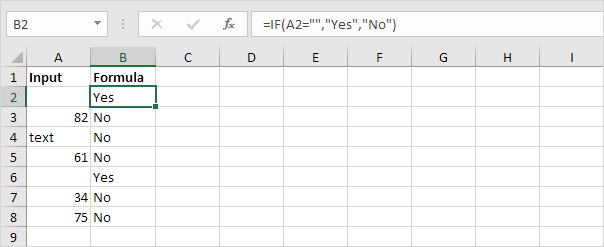Note: if the input cell contains a space, it looks blank. However, if this is the case, the input value is not equal to an empty string and the IF function above will return No.

2. Use IF and ISBLANK to produce the exact same result.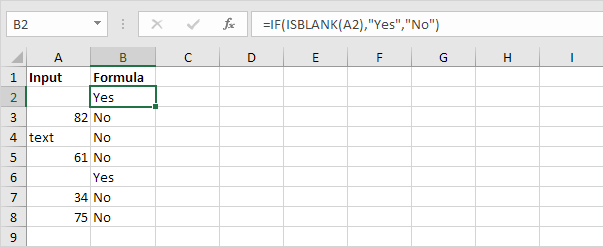Note: the ISBLANK function returns TRUE if a cell is empty and FALSE if not. If the input cell contains a space or a formula that returns an empty string, it looks blank. However, if this is the case, the input cell is not empty and the formula above will return No.

### If Not Blank

In Excel, <> means not equal to.

1. The IF function below multiplies the input value by 2 if the input value is not equal to an empty string (two double quotes with nothing in between), else it returns an empty string.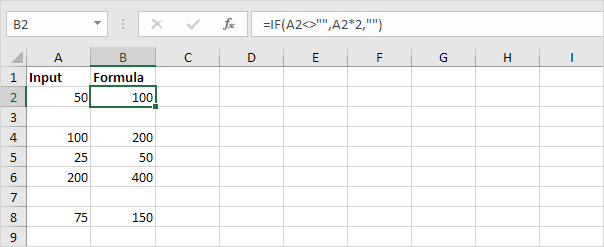2. Use IF, NOT and ISBLANK to produce the exact same result.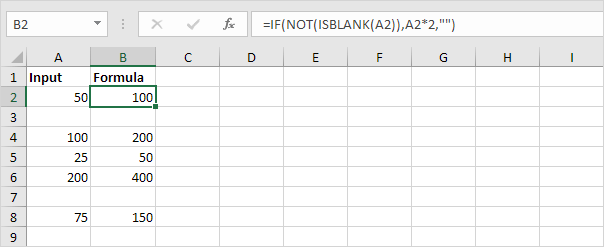### Highlight Blank Cells

You can use conditional formatting in Excel to highlight cells that are blank.

1. For example, select the range A1:H8.2. On the Home tab, in the Styles group, click Conditional Formatting.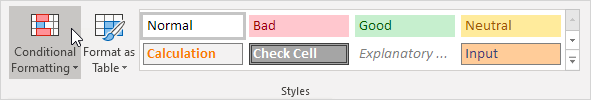3. Click Highlight Cells Rules, More Rules.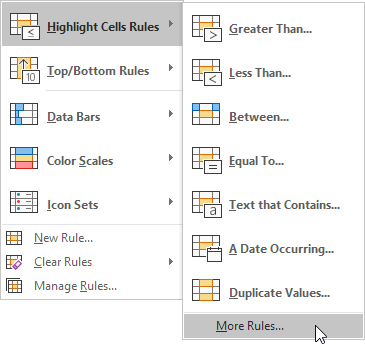4. Select Blanks from the drop-down list, select a formatting style and click OK.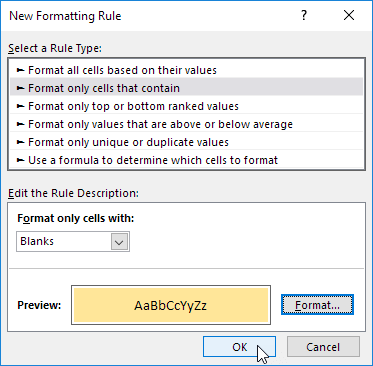Result.